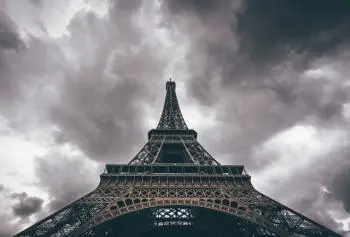# What Are Vertices? Relationship with Edges and ExamplesGeometry, as a fundamental branch of mathematics, allows us to understand and analyze the forms and structures that surround us. In this article, we will delve into the fascinating world of vertices in geometry and explore their importance in the description and classification of geometric figures.

A vertex, that meeting point where the sides of a figure converge, is much more than a simple intersection. It is a key concept that gives us valuable information about the shape, angles, and inherent properties of geometric figures. From triangles to more complex polygons, each figure has a specific number of vertices that influence its behavior and distinctive features.

## Vertex Definition

A vertex is an intersection point or a union point in a geometric figure or in a network. In mathematics, the term "vertex" is used in different contexts, depending on the type of figure or structure being considered. Here are some common definitions of vertex in different disciplines:

In plane geometry, a vertex refers to a point where two or more lines, segments, rays, or angles meet. For example, in a triangle, the vertices are the points where the three sides intersect. In a square or rectangle, the vertices are the points where the four angles meet.

In three-dimensional geometry, vertices are the points where the edges of a polyhedron, such as a cube, prism, or tetrahedron, meet. Each vertex is defined by three or more edges that converge at that point.

## Examples of Vertices on a Day-to-day Basis

In everyday life, you can find examples of vertices in different situations. Here are some common examples:

1. Corners of a room: The corners where the walls of a room meet are vertices. Each corner represents the point of intersection between two walls and a ceiling or a floor.

2. Street intersection: Street junctions or intersections are examples of vertices in the context of a road network. Each intersection is a meeting point between two or more streets.

3. Meeting sides in a book: When you close a book, the points where the sides of the pages meet form vertices.

4. Points of a traffic triangle: Traffic triangles that are placed on highways to indicate an emergency situation have three vertices at their corners.

5. Meeting of tracks on a railway: On railways, the points where two or more tracks intersect are vertices. These intersection points allow trains to change direction or follow different routes.

6. Electrical Connections: In an electrical circuit, the connection points where wires or electronic components meet form vertices. In electricity, these points are known as nodes and Kirchhoff's laws allow us to study the passage of electric current through them.

7. Hot air balloons: in a hot air balloon, the basket is attached to the balloon by the vertices.

8. The Eiffel Tower: the highest point of the Eiffel Tower is the top vertex of a quadrangular pyramid.

## Examples of Flat Geometric Figures with Their Number of Vertices

 Figure vertices edges Definition Point 0 0 A point has no dimensions, edges, or vertices. Line 2 1 A line has infinitely many points and is defined by two endpoints. Triangle 3 3 It has three sides and the sum of its interior angles is 180°. Square 4 4 A square is a polygon with four equal sides and four vertices. Rectangle 4 4 This figure is a polygon with four sides with right angles. Pentagon 5 5 A pentagon is a polygon with five sides and five vertices. Hexagon 6 6 A hexagon is a polygon with six sides. Circle 0 0 A circle has no sides. It is a closed curve with a center.
Author:

Published: June 2, 2023
Last review: June 2, 2023